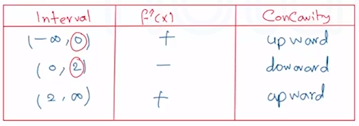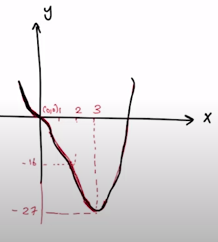Need Help?

Subscribe to Calculus A

###### \${selected_topic_name}
• Notes

Discuss the curve $y=x^{4}-4 x^{3}$ with respect to concavity, points of inflection
and local maximum and minimum.Use this information to sketch the curve

$f(x)=x^{4}-4 x^{3}$
$f^{\prime}(x)=4 x^{3}-12 x^{2}$
$f^{\prime \prime}(x)=12 x^{2}-24 x$

(1) To find Critical numbarse $\rightarrow f^{\prime}(x)=0$

$4 x^{3}-12 x^{2}=0 \rightarrow 4 x^{2}(x-3)=0$

$x=0, x=3$

$f(0)=0^{4}-4(0)^{3}=0$ local min
$f(3)=(3)^{4}-4(3)^{3}=-27$

$f^{\prime \prime}(0)=0$
$f^{\prime \prime}(3)=36>0$
$f^{\prime}(3)=0$

\ $f(3)=-27$ is alocal min

$f^{\prime \prime}(x)=12 x^{2}-24 x$

$12 x^{2}-24 x=0 \longrightarrow 12 x(x-2)=0$

$x=0 \quad,\quad x=2$$(0,0)$ Inflection point
$(2,-16)$ Inflection point

$(3,-27)$
$(0,0)$
$(2,-16)$